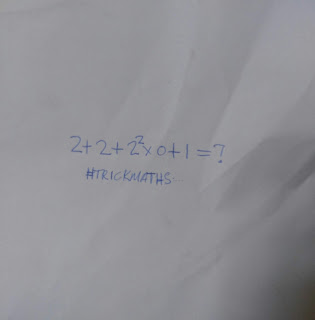# Viral Math Quiz 2 + 2 + 2square × 0 + 1 = ? Can you solve?

Viral Math Quiz 2 + 2 + 2square × 0 + 1 = ? Can you solve?

This Math Quiz has gone viral on social media and many people failed it. If this is your first time of seeing it, then what is your answer? Try to solve it and compare with our own solving  bellow.

The Quiz is 2 +2 + 2square × 0 + 1 = ?SOLUTION
The first thing to do is to solve the exponent according to PEMDAS.
So, 2 square = 2 × 2 = 4.

Secondly, we multiply.
That is 4 × 0 = 0.

Finally we add. 2 + 2 + 0 + 1 = ?
Emplies 5 = ?.